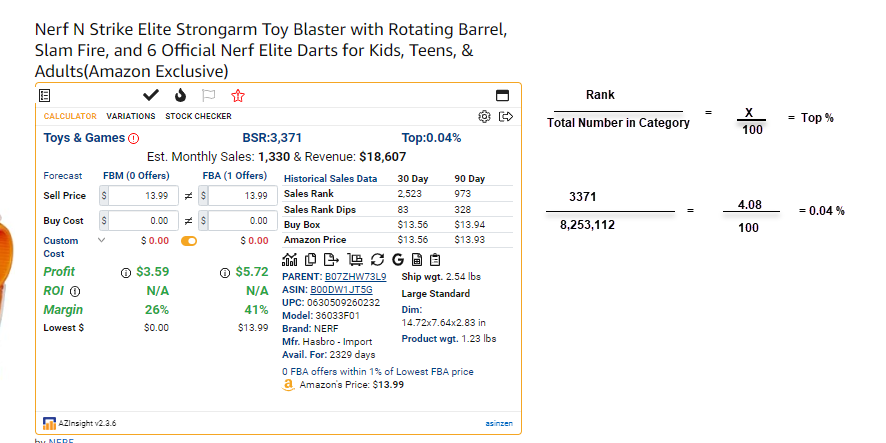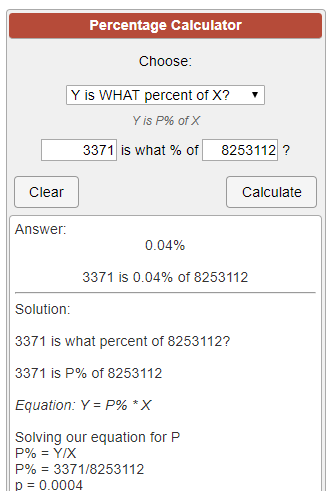# How is the Top % calculated?

The top % is calculated from data provided by Keepa.com. The method used to calculate the Top % is by taking the current BSR (rank) of the ASIN and then dividing it by the total number of items found in that amazon category according to Keepa, then converting it to a percent by diving by 100.

For example: If the Amazon category Toys and Games has a total of 8,253,112 products (according to Keepa) and the Current BSR of the ASIN you are looking at is 3,371, then the Top % of that product is 0.04%. Below is the breakdown of how to get this number.Reference: You can use https://www.calculatorsoup.com/calculators/math/percentage.php and select Y is What Percent of X